Test: Problems on Similar Triangles

# Test: Problems on Similar Triangles - Class 10

Test Description

## 10 Questions MCQ Test Mathematics (Maths) Class 10 - Test: Problems on Similar Triangles

Test: Problems on Similar Triangles for Class 10 2023 is part of Mathematics (Maths) Class 10 preparation. The Test: Problems on Similar Triangles questions and answers have been prepared according to the Class 10 exam syllabus.The Test: Problems on Similar Triangles MCQs are made for Class 10 2023 Exam. Find important definitions, questions, notes, meanings, examples, exercises, MCQs and online tests for Test: Problems on Similar Triangles below.
Solutions of Test: Problems on Similar Triangles questions in English are available as part of our Mathematics (Maths) Class 10 for Class 10 & Test: Problems on Similar Triangles solutions in Hindi for Mathematics (Maths) Class 10 course. Download more important topics, notes, lectures and mock test series for Class 10 Exam by signing up for free. Attempt Test: Problems on Similar Triangles | 10 questions in 10 minutes | Mock test for Class 10 preparation | Free important questions MCQ to study Mathematics (Maths) Class 10 for Class 10 Exam | Download free PDF with solutions
 1 Crore+ students have signed up on EduRev. Have you?
Test: Problems on Similar Triangles - Question 1

### Consider the triangle shown in the figure where BC = 12 cm, Db = 9 cm, CD = 6 cm and What is the ratio of the perimeter of the triangle ADC to that of the triangle BDC?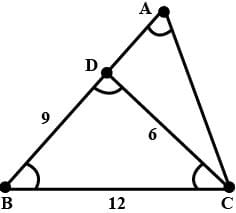Detailed Solution for Test: Problems on Similar Triangles - Question 1

Here, ∠ACB = c+180-(2c-b) = 180-(b+c)
So, We can say that Δ BCD and &delta ABC will be similar.
According to property of similarity,
AB/12 = 12/9
Hence,
AB = 16
AC/6 = 12/9
AC = 8
Hence, AD = 7 and AC = 8
Now,
Perimeter of Δ ADC / Perimeter of ΔBDC,
= (6+7+8)/(9+6+12)
= 21/27 = 7/9.

Test: Problems on Similar Triangles - Question 2

### In ΔPQR, ∠P = 60°, ∠Q = 50°. Which side of the triangle is the longest ?

Test: Problems on Similar Triangles - Question 3

### If ABC and PQR are similar triangles in which ∠ A = 470 and ∠ Q = 830, then ∠ C is:

Test: Problems on Similar Triangles - Question 4

In the given figure, T and B are right angles. If the lengths of AT, BC and AS (in centimeters) are 15, 16 and 17 respectively, then the length of TC (in centimeters) is: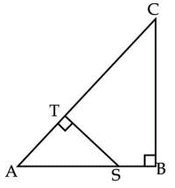Detailed Solution for Test: Problems on Similar Triangles - Question 4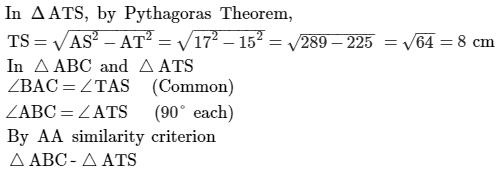Test: Problems on Similar Triangles - Question 5

In the adjoining figure, AD = 2 cm, DB = 3 cm, AE = 5 cm and DE || BC, then find EC.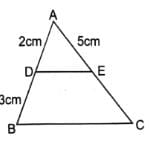Test: Problems on Similar Triangles - Question 6

In an equilateral triangle ABC, if AD ⊥ BC. Then​

Detailed Solution for Test: Problems on Similar Triangles - Question 6

∆ ABC, in which sides are AB=BC= AC= a units and AD is perpendicular to BC ,

AB²= AD²+ BD²     (by Pythagoras theorem)

a² = AD² + (a/2)²   [BD= 1/2BC, since in an equilateral triangle altitude AD is  perpendicular bisector of BC ]

⇒ 3AB²/4= AD²               [ AB= a]

Test: Problems on Similar Triangles - Question 7

In two triangles ABC and PQR,Given that ∠A = ∠R and ∠B = ∠Q, which of the following is true?​

Detailed Solution for Test: Problems on Similar Triangles - Question 7

∠A = ∠R and ∠B = ∠Q
The names of triangles are written in such a manner in which the Angles are correspondingly equal which means that the vertices at which the angles of the two triangles are equal is placed accordingly. So since the the angle A and Angle R are equal and Angle B and Angle D are equal we have ΔABC - ΔRQP

Test: Problems on Similar Triangles - Question 8

Triangles ABC, DEF are similar, ∠A = 75° , ∠B = 85° so ∠F = ?​

Test: Problems on Similar Triangles - Question 9

A vertical stick 30 m long casts a shadow 15 m long on the ground. At the same time, a tower casts a shadow 75 m long on the ground. The height of the tower is:​

Detailed Solution for Test: Problems on Similar Triangles - Question 9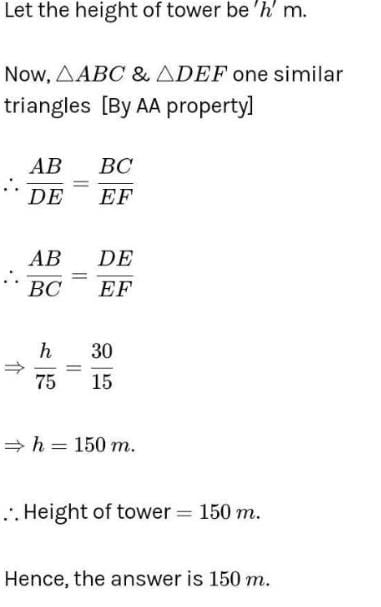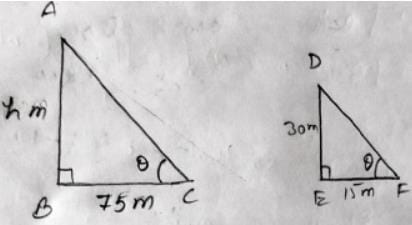Test: Problems on Similar Triangles - Question 10

Given two triangles ABC and PQR such that, AB = 2 cm, PQ = 3cm, ∠B = ∠Q BC = 5 cm, QR = 7.5 cm. AG and PS are medians . Find AG/PS = ?​

## Mathematics (Maths) Class 10

115 videos|478 docs|129 tests
Information about Test: Problems on Similar Triangles Page
In this test you can find the Exam questions for Test: Problems on Similar Triangles solved & explained in the simplest way possible. Besides giving Questions and answers for Test: Problems on Similar Triangles, EduRev gives you an ample number of Online tests for practice

## Mathematics (Maths) Class 10

115 videos|478 docs|129 tests